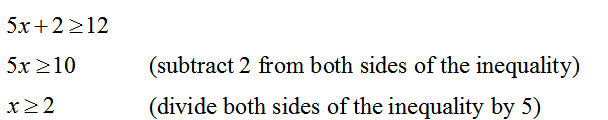# Two-step inequalities

Two-step inequalities are to one-step inequalities what two-step equations are to one-step equations – the same thing, but a bit more work is needed in order to solve them. Two-step inequalities are very easy to solve and the rules you have to adhere to are the same as for the one-step inequalities – remember that you need to change the sign of inequality when multiplying or dividing the whole inequality with a negative number. The order of operations will not come into play often since there are not many operations to perform here.

We will now show you on this example how this inequality looks like and what it takes to solve it:

4x + 8 > 20

The first thing you need to do is to put all the numbers on the right side and the variables on the left. Then perform the additions and subtractions. Like this:

4x > 20 – 8

4x > 12

The only thing left to do now is to divide the whole inequality by 4 to get the final, simplified result. Since the number we are dividing the inequality by is positive, it is not necessary to change the sign of inequality. Therefore:

4x > 12 |:4

x > 3And now we have the final result. If you wish to practice solving two-step inequalities a bit more, please feel free to use the worksheets below.

## Two-step inequalities exams for teachers

 Exam Name File Size Downloads Upload date Integers Two-step inequalities – Integers – very easy 0 B 11188 January 1, 1970 Two-step inequalities – Integers – easy 0 B 10970 January 1, 1970 Two-step inequalities – Integers – medium 0 B 16266 January 1, 1970 Two-step inequalities – Integers – hard 0 B 6464 January 1, 1970 Two-step inequalities – Integers – very hard 0 B 4586 January 1, 1970 Decimals Two-step inequalities – Decimals – very easy 0 B 2635 January 1, 1970 Two-step inequalities – Decimals – easy 0 B 2199 January 1, 1970 Two-step inequalities – Decimals – medium 0 B 2266 January 1, 1970 Two-step inequalities – Decimals – hard 0 B 1471 January 1, 1970 Two-step inequalities – Decimals – very hard 0 B 1286 January 1, 1970 Fractions Two-step inequalities – Fractions – very easy 0 B 3837 January 1, 1970 Two-step inequalities – Fractions – easy 0 B 2953 January 1, 1970 Two-step inequalities – Fractions – medium 0 B 3245 January 1, 1970 Two-step inequalities – Fractions – hard 0 B 1982 January 1, 1970 Two-step inequalities – Fractions – very hard 0 B 2048 January 1, 1970

## Two-step inequalities worksheets for students

 Worksheet Name File Size Downloads Upload date Two-step inequalities – Integers 0 B 7836 January 1, 1970 Two-step inequalities – Decimals 0 B 2375 January 1, 1970 Two-step inequalities – Fractions 0 B 2627 January 1, 1970